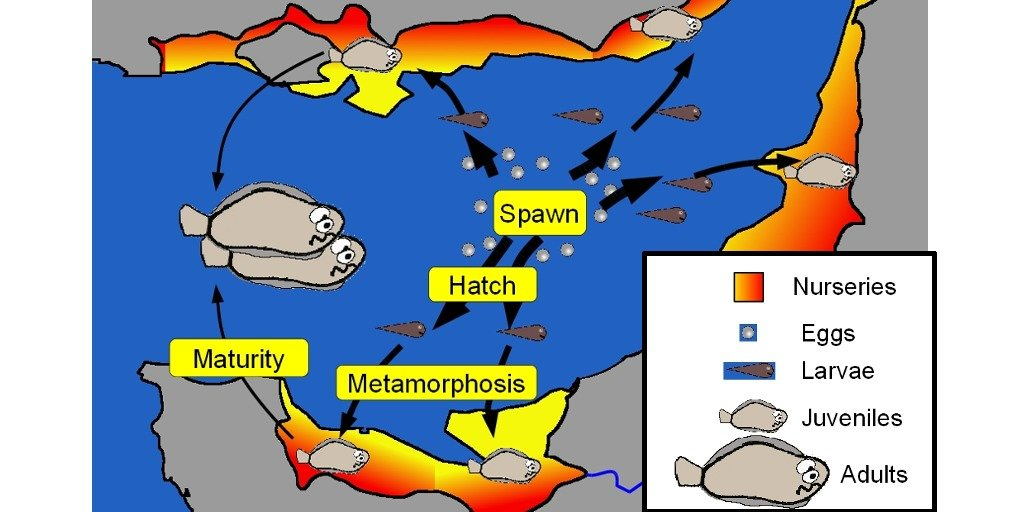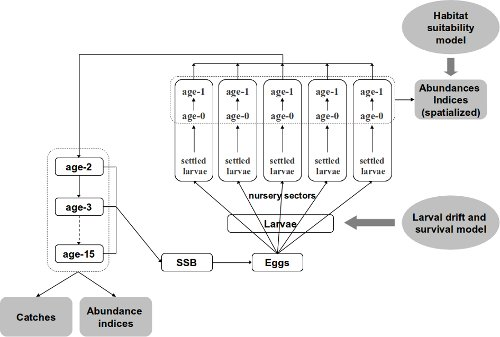# Bayesian life cycle model: OpenBUGS code

## Summary

The `OpenBUGS` code presented below is the additional material of a publication. This is the life cycle model of a marine species (the sole in the Eastern Channel). The Bayesian hierarchical model incorporates indices of juvenile spatialized abundance, larval dispersion, adult abundance indices and catches by fishery.

## Publication associated

Rochette Sebastien, Le Pape Olivier, Vigneau Joel, Rivot Etienne (2013). A hierarchical Bayesian model for embedding larval drift and habitat models in integrated life cycles for exploited fish. Ecological Applications, 23(7), 1659-1676. Publisher’s official version : http://dx.doi.org/10.1890/12-0336.1 , Open Access version : http://archimer.ifremer.fr/doc/00161/27243/Figure 1: DAG of the Bayesian life cycle model

### BUGS code to estimate parameters of a full life cycle model

1. Outputs of an individual-based model for larval drift and survival that provided yearly estimates of the dispersion and mortality of eggs and larvae, from spawning grounds to settlement in several coastal nurseries;
2. A habitat suitability model, based on juvenile trawl surveys coupled with a geographic information system, to estimate juvenile densities and surface areas of suitable juvenile habitat in each nursery sector;
3. A statistical catch-at-age model for the estimation of the numbers-at-age and the fishing mortality on subadults and adults.

The following BUGS code refers to a specific scenario of the larvae to juvenile mortality process tested in the associated paper (see paper). This scenario (R3) assumes a density-independent mortality parameter common to all nurseries (a_BH) and a nursery-specific density-dependent mortality parameter (b_BH).

• Programmer: Sébastien Rochette, August 2012
• OpenBUGS version : 3.2.2

### Inputs

• max.a: Number of age classes
• max.t: Number of years
• max.Area: Number of nurseries
• obsJuvtime : Date of observation of juvenile indices (in months)
• A_eggs, B_eggs : Fecondity parameters (at age) as Fec = exp(A_eggs + B_eggs * log(Weight-at-age))
• Pf: Female proportion (at age)
• M0: Mortality between Age 0 & Age 1 (on each area)
• M: Natural mortality (at age)
• Dij: Matrix of larval repartition among (i) Total mortality and (ii) all the areas (for each year).
• Sstar: Surface area of each area
• W_stock: Matrix of individual weights-at-age for each year
• C_obs: Matrix of observed catches-at-age for each year from age 1 to age 10
• C_obs11Plus: Vector of observed catches-at-age for each year summed for ages 11 and more
• IA_BTS_obs: Matrix of observed adult abundance indices for age 2 to max.a, for each year.
• IA_Age0: Matrix of observed age-0 abundance indices, calculated from a habitat suitability model for each year and each area.
• IA_Age1: Matrix of observed age-1 abundance indices, calculated from a habitat suitability model for each year and each area.

### BUGS code:

``````# Bayesian Life Cycle model of a fish species
# website: http://statnmap.com

model
{

# ----------------------------
# Priors
# -----------------------------

# Catchability of BTS abundance indices (large priors)
# Common Q for a >= 2
Logq_0 ~ dunif(-10,10)
Logq_1 ~ dunif(-10,10)
Logq_AgeSup ~ dunif(-10,10)
q_0 <- exp(Logq_0)
q_1 <- exp(Logq_1)
q_AgeSup <- exp(Logq_AgeSup)

# Fishing mortality = Selectivity * Effort
# Effort (gamma prior)
E_Eff1 ~ dunif(0.00001, 10)
CV_Eff ~ dunif(0.00001, 0.99999)
a_Eff <- 1 / (CV_Eff*CV_Eff)
b_Eff <- (1 / (CV_Eff*CV_Eff)) * (1/E_Eff1)
for(t in 1:max.t)
{
Effort[t] ~ dgamma(a_Eff, b_Eff)
}

# Age of changing Selectivity slope (gamma prior)
# Logistic
CV_Selec <- 0.9
a_Selec <- 1 / (CV_Selec * CV_Selec)
bAge50_S <- (1 / (CV_Selec*CV_Selec)) * (1/E_Age50_S)
bDelta_S <- (1 / (CV_Selec*CV_Selec)) * (1/E_Delta_S)
Age50_S ~ dgamma(a_Selec,bAge50_S)
Delta_S ~ dgamma(a_Selec,bDelta_S)
for(a in 1:max.a)
{
Selec_p[a] <- (exp((2*Age50_S*log(3)/Delta_S)*(a/Age50_S-1))/(1+(exp((2*Age50_S*log(3)/Delta_S)*(a/Age50_S-1)))) )
Selec[a] <- Selec_p[a] / Selec_p[max.a]
}
# Induced selectivity for abundance indices surveys
for(a in 1:max.a) {
SelecQ[a] <- cut(Selec[a])
q[a] <- max(q_AgeSup * SelecQ[a] ,1.0E-7)
}

#Combined F=Selec*Effort (gamma prior)
CV_F ~ dunif(0.00001, 0.99999)
a_F <- 1 / (CV_F*CV_F)
for(t in 1:max.t)
{
for(a in 1:max.a)
{
mean_F[a,t] <- Selec[a] * Effort[t]
b_F[a , t] <- (1 / (CV_F*CV_F)) * (1/mean_F[a,t])
F[a,t] ~ dgamma(a_F, b_F[a, t])
}
# Parameter of interest : Fishing mortality
F3_8[t] <- mean(F[3:8,t])
}

# Stock-Recruitment (SR) : Beverton & Holt
#Common a_BH & Hierarchical b_BH
mean_aBH ~ dunif(0.00001, 4)
CV_aBH ~ dunif(0.00001, 1)
a_aBH <- 1/(CV_aBH*CV_aBH)
b_aBH <- (1/(CV_aBH*CV_aBH))*(1/mean_aBH)
a_BH ~ dgamma(a_aBH,b_aBH)

mean_bBH ~ dunif(0.00001, 0.1)
CV_bBH ~ dunif(0.00001, 3.5)
a_bBH <- 1/(CV_bBH*CV_bBH)
b_bBH <- (1/(CV_bBH*CV_bBH))*(1/mean_bBH)

# Induced Psi & K
Psi <- exp(-a_BH)
for(Area in 1:max.Area){
b_BH[Area] ~ dgamma(a_bBH,b_bBH)
K[Area] <- a_BH/b_BH[Area] * 1/(exp(a_BH) -1)
}

# Process error on SR (large prior)
log_sigma2_SR ~ dunif(-10,10)
sigma2_SR <- exp(log_sigma2_SR)
Tau_SR <- 1 / sigma2_SR

# Initialisation of number of Age 1 on Areas for year 1
# large priors according to what expected while looking at ICES data (sum(N1)<1.2E5)
for(Area in 1:max.Area)
{
N1[Area,1] ~ dunif(1.0E-7, 1.0E7) # Cannot be null for cohort depletion
}

# Initialisation of the number at all ages for year 1 (x1000)
# For age 1
mean_logN1_NoSpace <- log(sum(N1[,1]))
Na1 ~ dlnorm(mean_logN1_NoSpace, Tau_P)
N[1, 1] <- Na1
# P will be N/N[age=1,cohort] to facilitate convergence of MCMC
P[1,1] <- 1
# For age 2 to max.a
for(a in 2:max.a)
{
Na1[a] ~ dunif(1.0E-7, 1.0E7) # Cannot be null for cohort depletion
N[a,1] <- Na1[a]
P[a, 1] <- 1
}

# Variable larval key repartition
# Prior on the parameters of the Dirichlet distribution
log.p.dirich[1:k] ~ dmnorm(mu.log.p[],Tau[,])
for(i in 1:k) { p.dirich[i] <- exp(log.p.dirich[i]) } # must be positive
# Likelihood to learn from the data Dij (14/27 years available)
for (t in 1:max.t) { Dij[t,1:k] ~ ddirich(p.dirich[1:k]) }

# ----------------------------
# Population dynamics
# ----------------------------

# "Artificial" Process error on dynamic equations (to speed up the sampling process)
# (correponds to a Normal variability in the natural mortality)
Tau_P <- 1000

for(t in 1:max.t)
{
# Spatialisation for Larvae, Age 0 and Age 1
for(Area in 1:max.Area)
{
# Repartition of Eggs to larvae with the larval key repartition
Larvae[Area, t] <- Eggs[t] * Dij[t,Area]

# Berverton&Holt recruitment function --> age 0
# R = K * S / ( So + S)
#Sstar = Surface of nurseries
L_star[Area,t] <- Larvae[Area,t] / Sstar[Area]
mean_N0[Area,t] <- (Psi * K[Area] * L_star[Area,t]) / (K[Area] + Psi * L_star[Area,t]) * Sstar[Area]

# log-normal error on Age-0 juveniles survival
mean_logN0[Area, t] <- log(mean_N0[Area, t])
LogN0[Area, t] ~ dnorm(mean_logN0[Area, t], Tau_SR)
N0[Area, t] <- exp(LogN0[Area, t])

# Survival to age 1 (quasi deterministic)
mean_LogN1[Area, t+1] <- log(N0[Area, t] * exp(-M0[Area] * (1-(obsJuvtime/12))))
N1[Area, t+1] ~ dlnorm(mean_LogN1[Area, t+1], Tau_P)
} # End of Area loop

# Sum age 1 over space (quasi deterministic)
mean_logN1_NoSpace[t+1] <- log(sum(N1[,t+1]))
N[1, t+1] ~ dlnorm(mean_logN1_NoSpace[t+1], Tau_P)
# P will be N/N[age=1,cohort] to facilitate convergence of MCMC
# such that all P[1,] = 1
P[1, t+1] <- 1

# Dynamic equation - Age 1 : max
# (quasi deterministic)

# Calculation of cohorts number
for(a in 1:(max.a))
{
Row[a, t] <- step(t - a - 1) * (1) + step(a - t) * (a - t +1)
Col[a, t] <- step(t - a - 1) * (t - a + 1) + step(a - t) * (1)
}

# Cohort depletion
# P is N/N[age=1,cohort] to facilitate convergence of MCMC
for(a in 1:(max.a-1))
{
log_mean_P[a + 1, t + 1] <- log(P[a,t] * exp(-M[a]-F[a,t]) )
P[a + 1, t + 1] ~ dlnorm(log_mean_P[a + 1, t + 1], Tau_P)
N[a +1 , t +1] <- P[a +1, t +1] * N[Row[a, t], Col[a, t]]
}

# Fecundity (relation weight-at-age <-> number of eggs)
# (W_Stock = weight-at-age in data)
for(a in 1:max.a)
{
Fec[a, t] <- exp(A_eggs + B_eggs * log(W_Stock[a, t] * 1000))
}

# SSB : Only > age 3 participate to SSB
for(a in 1:max.a)
{
W_Nb[a, t] <- N[a, t] * W_Stock[a, t] * step(a - 3)
}
# Parameter of interest
SSB[t] <- sum(W_Nb[,t])

# Number of eggs (x 1000) (After a P_Period mortality ~ spawning period)
# Only > age 3 participate to reproduction
for(a in 1:max.a)
{
Nb_eggs[a, t] <- N[a, t] * exp(P_Period[t]*(-M[a]-F[a,t])) * Pf[a] * Fec[a,t] * step(a - 3)
}

} # end of loop on t

# For predictions on max.t+1, we use catches and fecundity of the previous year
for(a in 1:max.a)
{
Nb_eggs[a, (max.t + 1)] <- N[a, (max.t + 1)] * exp(P_Period[max.t]*(-M[a]-F[a,max.t])) * Pf[a] * Fec[a,max.t] * step(a - 3)
}

# ----------------------------
# Observation equations
# ----------------------------
# Observation variance
# Catches (observation variance fixed)
# USE OF EXPERTIZE HERE
# CV_C <- 0.2 #in data [sensitivity tested 0.1 ; 0.2 ; 0.4]
sigma2LogC <- log(CV_C*CV_C+1)
tauLogC <- 1/sigma2LogC
# Under declaration [not used]
for (a in 1:max.a) { under_declare[a] <- 1 }

# Abundance Indices
CV_IA <- sqrt(exp(sigma2LogIA)-1)
Logsigma2LogIA ~ dunif(-10,10)
sigma2LogIA <- exp(Logsigma2LogIA)
tauLogIA <- 1/sigma2LogIA
# Juveniles
CV_IAJuv <- sqrt(exp(sigma2LogIAJuv)-1)
Logsigma2LogIAJuv ~ dunif(-10,10)
sigma2LogIAJuv <- exp(Logsigma2LogIAJuv)
taulogIAJuv <- 1/sigma2LogIAJuv

# Observations
# Catches (X 1000)
for(t in 1:(max.t)) {
for(a in 1:max.a) {
C_mean[a, t] <- under_declare[a] * (
N[a, t] * ( F[a, t] / (F[a, t] + M[a])) * (1 - exp(-1 * (M[a] + F[a, t]))) )
C_meancor[a, t] <- C_mean[a,t] * exp(- 0.5*sigma2LogC)
logCmean[a,t] <- log(C_meancor[a,t])

}
for(a in 1:10){
C_obs[a,t] ~ dlnorm(logCmean[a,t], tauLogC)
}
# Observations of catches for age>10 grouped in a 10+ group
C_mean11Plus[t] <- sum(C_meancor[11:max.a,t])
logC_mean11Plus[t] <- log(C_mean11Plus[t])
C_obs11Plus[t] ~ dlnorm(logC_mean11Plus[t], tauLogC)
}

for(t in 1:(max.t)) {
for(a in 2:max.a) {
#Abundance indices including selectivity of the fishery
#SelecQ[a] included in q[a]
log_IA_BTS[a, t] <- log(q[a] * N[a, t])
IA_BTS_obs[a, t] ~ dlnorm(log_IA_BTS[a, t], tauLogIA)
}
}

# Abundance for juveniles
for(Area in 1:max.Area){
for(t in 1:max.t){
# Estimation of N0 is at observation time with Dij
log_N0[Area, t] <- log(q_0 * N0[Area, t])
IA_Age0[Area, t] ~ dlnorm(log_N0[Area,t], taulogIAJuv)
# Estimation of N1 is in January whereas observation is in September
log_N1[Area, t] <- log(q_1 * N1[Area,t] * exp(-(M+F[1,t])*(obsJuvtime/12)))
IA_Age1[Area,t] ~ dlnorm(log_N1[Area,t], taulogIAJuv)
}
}

} #End of model``````

###### Citation:

``` Rochette Sébastien. (2015, Jul. 23). "Bayesian life cycle model: OpenBUGS code". Retrieved from https://statnmap.com/2015-07-23-bayesian-life-cycle-model-openbugs-code/. ```
``````@misc{Roche2015Bayes,